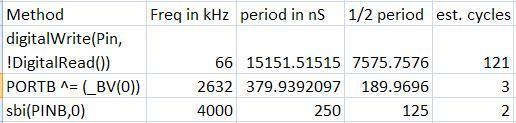# Ready, Set, Oscillate! The Fastest Way to Change Arduino Pins

##### 18 Aug 2010There are many ways to change an output pin. The way we know and love is the famous digitalWrite() function. (Spoiler: Want a faster digitalWrite? Download Here!)

But even the Arduino Reference claims that it is not the most efficient. The Arduino functions do a lot of error checking to make sure the pin is configured right and has to map Arduino numbering to actual IO ports.  All this cost processor cycles, and time.  But how much? This article is not to teach you how to useIO registers, you can read about it on the Arduino Port Manipulation page. This is to cover exactly how inefficient the Arduino functions are.

I ran some tests to find out. The test platform was a 16Mhz Arduino and a very nice oscilloscope watching one of its output pins. I ran two tests, one setting the pins to know values(on or off), and one that ‘flips’ the pin from its previous state.  My code was inside a never ending for loop, so the result would always be a square wave form that I could measure in frequency.

The estimated CPU cycles is calculated from ½ the waveform period measured divided by the period of 16Mhz, since it takes two write operations to complete a full period in a waveform.  There could be some differences in the machine instructions it takes to set a bit compared to clearing a bit, so this is somewhat rough.

The 3 methods I tested were

• digitalWrite(pin, LOW);         digitalWrite(pin, HIGH);
• CLR(PORTB, 0) ;     SET(PORTB, 0);
• PORTB |= _BV(0);                   PORTB &= ~(_BV(0));

The macros used:

#define CLR(x,y) (x&=(~(1<<y)))

#define SET(x,y) (x|=(1<<y))

#define _BV(bit) (1 << (bit))

The resultsAs you can see, digitalWrite takes around 56 cycles to complete, while direct Port addressing takes 2 cycles. That’s a big difference in time for programs that have lot’s of IO operations!

Next I tested just flipping a pin. By this I mean I just changed the pin state without knowing what the initial state was without testing. The methods of testing

• PORTB ^= (_BV(0))
• sbi(PINB,0)

#define sbi(port,bit) (port)|=(1<<(bit))

The resultsWow, the Arduino method takes a whopping 121 cycles to flip a pin! The sbi() using the PIN register is a neat trick for what usually is a read only register, and is the fastest at only 2 cycles.

So you see, the Arduino functions take much MUCH longer to complete pin operations then using direct port IO. But there is a reason why. Arduino does a lot of error checking, and has to look up pin number mappings to actual Atmega pins. Direct port access is not for the faint of heart, but it can be much faster for when you are ready to take off some of the Arduino training wheels.

Credit to Webbot for showing us the PIN register trick.UPDATE: Well, it seems the attention of my article has made me aware of a neat-o library for Arduino that keeps the code simple, but runs just as fast as direct port manipulation. Arduino Forum post here. I just tested the digitalWriteFast2() function and it also seems to only require 2 cycles to complete.